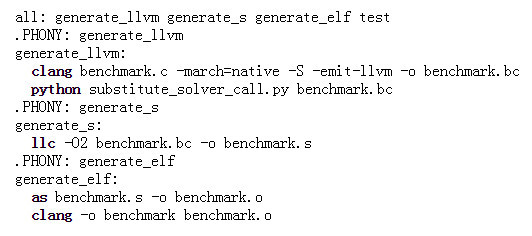# 都有 Python 了，还要什么编译器！

2019年02月19日 01:54来源：未知手机版

1. 汇编代码不可移植;

2. 虽然在现代工具的辅助下变得容易了些，但 Assembly 编程仍然需要大量繁琐的工作。

1. 使用 Clang 为基准生成 LLVM 中间代码，该基准用于测量 solve_5，一个不存在的函数;

2. 使 Python 在 LLVM 中生成线性求解器(linear solver)代码;

3. 使用 Python 脚本测试基准，用生成求解器替换 solve_5 调用;

4. 使用 LLVM 静态编译器将中间代码转换为机器代码;

5. 使用 GNU 汇编器和 Clang 的链接器将机器代码转换为可执行的二进制文件。Python 部分

# this generates n-solver in LLVM code with LLVMCode objects.

# No LLVM stuff yet, just completely Pythonic solution

defsolve_linear_system(a_array, b_array, x_array, n_value):

defa(i, j, n):

ifn == n_value:

returna_array[i * n_value + j]

returna(i, j, n+1)*a(n, n, n+1) - a(i, n, n+1)*a(n, j, n+1)

defb(i, n):

ifn == n_value:

returnb_array[i]

returna(n, n, n+1)*b(i, n+1) - a(i, n, n+1)*b(n, n+1)

defx(i):

d = b(i,i+1)

forjinrange(i):

d -= a(i, j, i+1) * x_array[j]

returnd / a(i, i, i+1)

forkinrange(n_value):

x_array[k] = x(k)

returnx_array

# this is basically the whole LLVM layer

I =

STACK = []

classLLVMCode:

# the only constructor for now is by double* instruction

def__init__(self, io, code = ):

self.io = io

self.code = code

def__getitem__(self, i):

global I, STACK

copy_code = % + str(I+1)

copy_code += = getelementptr inbounds double, double*

copy_code +=self.io + , i64 + str(i) + \n

copy_code += % + str(I+2)

copy_code += = load double, double* % + str(I+1)